Detalles de la revista
Formato
Revista
eISSN
2332-7774
Primera edición
30 Jan 2019
Calendario de la edición
2 veces al año
Idiomas
Inglés
Acceso abierto

# Damping of Thermocapillary Destabilization of a Liquid Film in Zero Gravity Through the Use of an Isothermal Porous Substrate

###### Páginas:35 - 42
Detalles de la revista
Formato
Revista
eISSN
2332-7774
Primera edición
30 Jan 2019
Calendario de la edición
2 veces al año
Idiomas
Inglés
INTRODUCTION

Dynamics of and patterning in thin liquid films are central to many important problems in engineering, geophysics, biophysics, etc. (Craster and Matar, 2008). A liquid film on an isothermal substrate is destabilized in zero gravity through the long wave instability of thermocapillary fingering. The lack of gravitational acceleration leads to quicker destabilization than under the influence of terrestrial or non-zero gravitational acceleration (Narendranath et al., 2014). A schematic of a liquid film on an isothermal solid substrate is depicted in Figure 1, along with some of the stabilizing and destabilizing effects.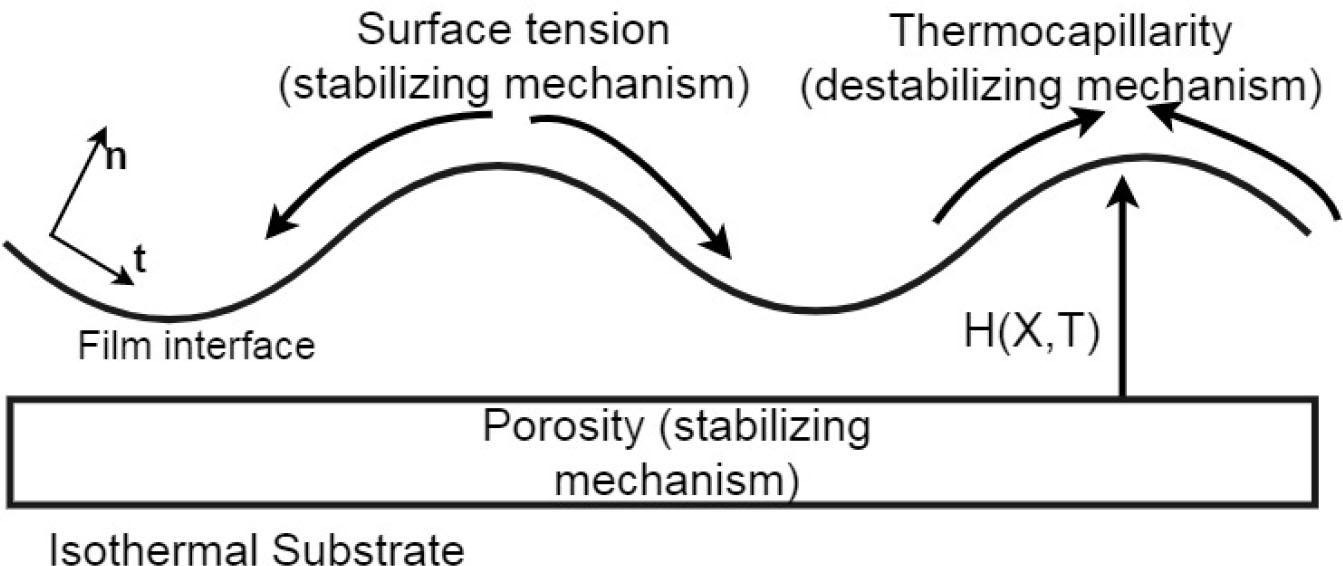Various stabilizing and destabilizing mechanisms that affect a liquid film on an isothermal solid substrate, where film is flat and parallel to the substrate. Periodic boundary conditions were used on the left and right edge. In this article, evaporation and concomitant vapor recoil effects were neglected.

A nonlinear evolution (partial differential) equation—derived from the Navier-Stokes equations and the energy conservation equation through a scaling and long wavelength approximation (Burelbach et al., 1988; Ajaev, 2012)—is solved numerically to study dynamics of liquid films in zero gravity (zero gravitational acceleration) and a porous substrate-induced stabilizing mechanism. This equation allows for the study of the influence of individual mechanisms by engaging required nonlinear terms through non-zero dimensionless coefficients (see Abbreviations). The rupture of liquid films (local thinning and exposure of underlying substrate) on isothermal substrates is governed by a balance between destabilizing thermocapillarity and stabilizing surface tension and gravity. In the absence of gravity, long wave instability-driven rupture occurs when thermocapillarity overwhelms surface tension (Narendranath et al., 2014).

Following the model put forth previously (Davis and Hocking,1999) or more recently (Liu et al., 2017), the effect of an isothermal porous substrate on zero-gravity evolution of non-evaporating liquid films, without disjoining pressures, was studied. Here, we only considered the case where the substrate was fully saturated with the liquid film and, hence, the effect of wettability; contact angles within the substrate and partial saturation on film dynamics were not considered. Evolution of spatial modes was visualized using recurrence plots and recurrence rate quantification. Other accounts in literature have studied an axisymmetric droplet spreading under the effect of injection or suction of fluid through a slot (Momoniat et al., 2010), the dynamic control of falling films through injection and suction (Thompson et al., 2016), and the spreading of droplets on a deep porous substrate (Liu et al., 2017). However, these did not account for the thermocapillary-driven instabilities in the absence of gravitational acceleration. The current study includes the effect of thermocapillarity, which was excluded from previous studies on films on porous substrates.

NONLINEAR EVOLUTION EQUATION

The dimensionless, nonlinear time evolution of a non-evaporating liquid film's interface thickness, H(H, T), in zero gravity is provided by the nonlinear partial differential Equation 6. A subscript signifies a partial derivative. The derivation of the evolution equation is summarized below. Inclusion of vital interfacial normal and shear stress balances has been discussed in greater detail in literature (Ajaev, 2012). Starting with the global mass conservation equation: $∂h∂t+∂Q∂x=w${{\partial h} \over {\partial t}} + {{\partial Q} \over {\partial x}} = w where quantities with dimensions h, x, t, and w are film thickness h=h(x,t), lateral coordinate, time, and percolation Darcy velocity, respectively. Q is the interfacial normal and shear stress balance leading to surface tension driven stabilization and thermocapillary led destabilization and fingering. $Q=σ3μ∂2h∂x2−γt2μh2∂T*i∂x$Q = {\sigma \over {3\mu }}{{{\partial ^2}h} \over {\partial {x^2}}} - {{\gamma t} \over {2\mu }}{h^2}{{\partial {T^{*i}}} \over {\partial x}}

The following scaling arguments are used to remove dimensionality from the governing equations of fluid dynamics in the lubrication approximation limit of small aspect ratio, $h0L≪1${\raise0.7ex\hbox{${{h_0}}$} \!\mathord{\left/ {\vphantom {{{h_0}} L}}\right.}\!\lower0.7ex\hbox{$L$}} \ll 1 . $x∼X h0Ca−1/3$x \sim X\,{h_0}\,C{a^{ - 1/3}}$t∼T tν$t \sim T\,{t_\nu }

Scaling the film thickness as h ~ Hh0 with leading order dimensionless interfacial temperature (Oron et al., 1997) given as $Ti=1(1+Bi H)${T^i} = {\raise0.7ex\hbox{$1$} \!\mathord{\left/ {\vphantom {1 {(1 + Bi\,H)}}}\right.}\!\lower0.7ex\hbox{${(1 + Bi\,H)}$}}$∂T*i∂x=∂∂x(ΔTTi+T∞)=ΔT∂Ti∂x=ΔT∂∂x(11+Bi H)${{\partial {T^{*i}}} \over {\partial x}} = {\partial \over {\partial x}}\left( {\Delta T{T^i} + {T_\infty }} \right) = \Delta T{{\partial {T^i}} \over {\partial x}} = \Delta T{\partial \over {\partial x}}\left( {{1 \over {1 + Bi\,H}}} \right)

In the derivation of the evolution equation (Equation 6), gravity-driven stabilization is neglected. Davis and Hocking (1999) use a surface tension time scale in their work. However, a viscous time scale is used to develop the evolution equation (Burelbach et al., 1988; Narendranath et al., 2014) to capture thermocapillary effects. Choice of a time scale based on a general characteristic velocity instead of a viscous scale yields the same evolution equation. $∂TH+[MaBiH2(1+BiH)2HX+SH3HXXX]X=NpmSHXX${\partial _T}H + {\left[ {MaBi{{{H^2}} \over {{{(1 + BiH)}^2}}}{H_X} + S{H^3}{H_{XXX}}} \right]_X} = {N_{pm}}S{H_{XX}}

In Equation 6, the term TH tracks the time evolution of the film interface. Thermocapillary interfacial instabilities are captured by the nonlinear term, $[MaBiH2(1+BiH)2]X${\left[ {MaBi{{{H^2}} \over {{{(1 + BiH)}^2}}}} \right]_X} . The stabilizing effect of surface tension is captured by [SH3HXXX]X. The capillary effect of a porous substrate, as modeled by the Darcy flow equation, is captured by the term NpmSHXX. The non-dimensional quantities Ma and Bi are the Marangoni and interface Biot number. The non-dimensional surface tension number is S, and the one-dimensional porosity of the substrate is captured in the non-dimensional number Npm. These non-dimensional numbers (see Abbreviations) are set to: Ma=5.00, Bi=1.00, S=100.0, which are values routinely used in literature (Oron, 2000). The magnitude of these values relative to each other determines film interface evolution. We have simulated the dynamics of dichloromethane films previously (Narendranath et al., 2014) with a Ma-S ratio (m of 0.1092. The current value of (m) of 0.05 leads to a slower thermocapillary evolution as compared to m =0.1092.

Equation 6 was solved with periodic boundary conditions, with a period of 2 π/k and a small perturbation initial condition, viz., 1 − 0.1cos(kx). Here, k was the fastest growing wavenumber (reciprocal of wavelength) as determined from a linear stability analysis, shown in Equation 7. $k=[1S{Ma(1+Bi)2−Npm}]0.5$k = {\left[ {{1 \over S}\left\{ {{{Ma} \over {{{(1 + Bi)}^2}}} - {N_{pm}}} \right\}} \right]^{0.5}}

The value of Npm is set to 0.001 to ensure very little divergence from the fastest growing wavelength for a porous substrate with respect to a non-porous substrate. This value of $NpmS=10−5${{{N_{pm}}} \over S} = {10^{ - 5}} ensures that initial film dynamics are strongly affected by the surface tension term as compared to capillarity due to the porous substrate. In the subsequent section, $NpmS=10−5${{{N_{pm}}} \over S} = {10^{ - 5}} is termed “weak porosity.”

The numerical solution was obtained using Wolfram Mathematica and its method of lines option along with the LSODA solver (Momoniat et al., 2010) and was validated with results in literature (Narendranath et al., 2014).

RESULTS
Dynamics of Film Evolution in Microgravity

Thermocapillary instabilities affecting a liquid film on an isothermal solid substrate (IS-sub) in zero gravity are shown in Figure 2. The film profile approached rupture due to thermocapillarity at approximately T=1650. In our simulations, rupture was considered to have been achieved when the local film thickness was ≈ 1000 times smaller (Narendranath et al., 2014) than the initial mean film thickness. Rupture of liquid films was caused by the development of higher frequencies that manifested as new thermocapillary spatial modes that evolved from the initial condition. When an isothermal porous substrate (IP-sub) was used in the simulations, the film persisted without rupturing until T=3500.0, at which point the simulation was halted, shown in Figure 3.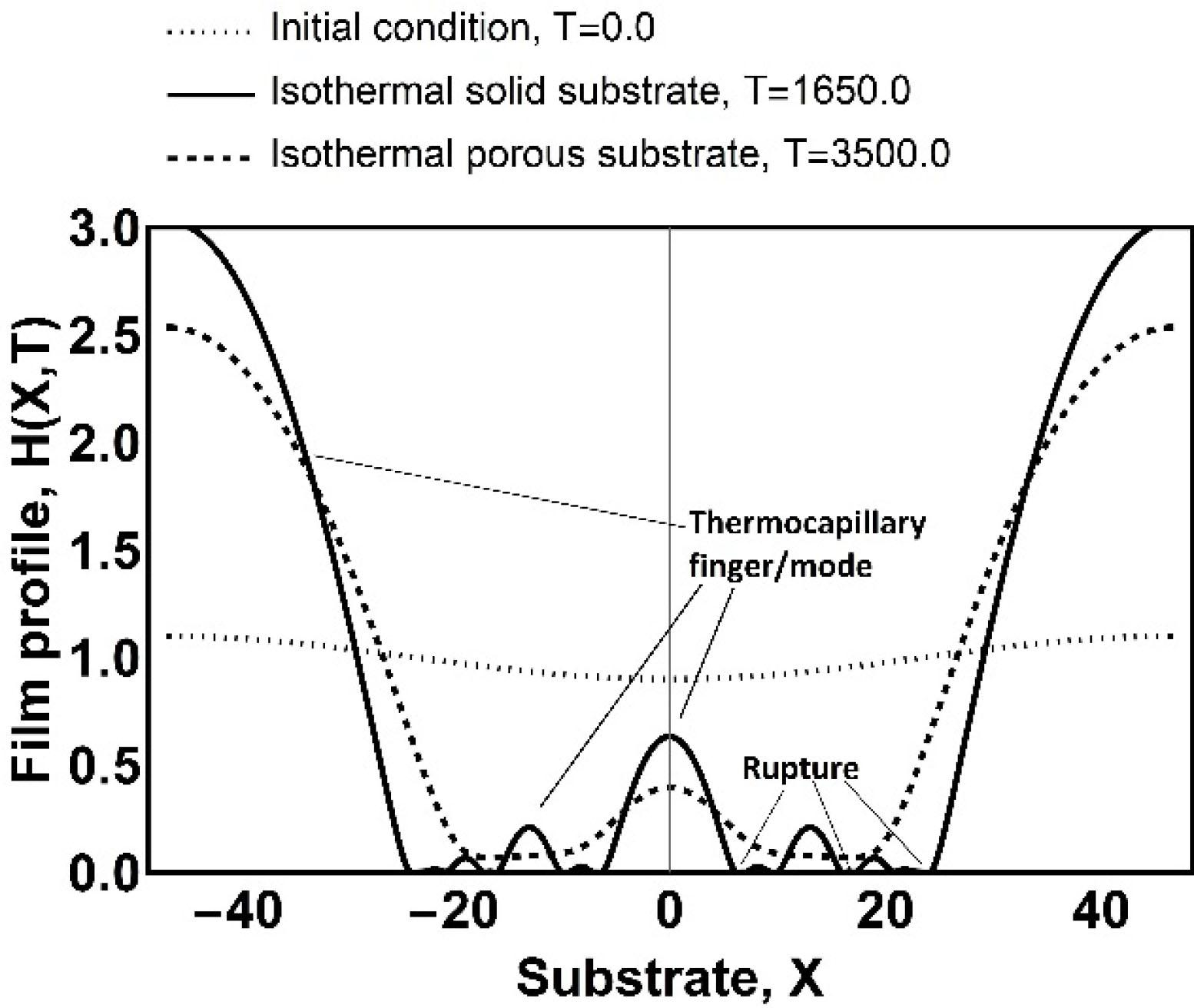Evolution of a liquid film towards thermocapillary-driven rupture from a cosine initial condition. An isothermal porous substrate allows for the film to persist without rupture for longer than with an isothermal solid substrate. T, time (dimensionless); X, span-wise coordinate (dimensionless); H(X,T), nonlinear time evolution of a non-evaporating liquid film's interface thickness (dimensionless).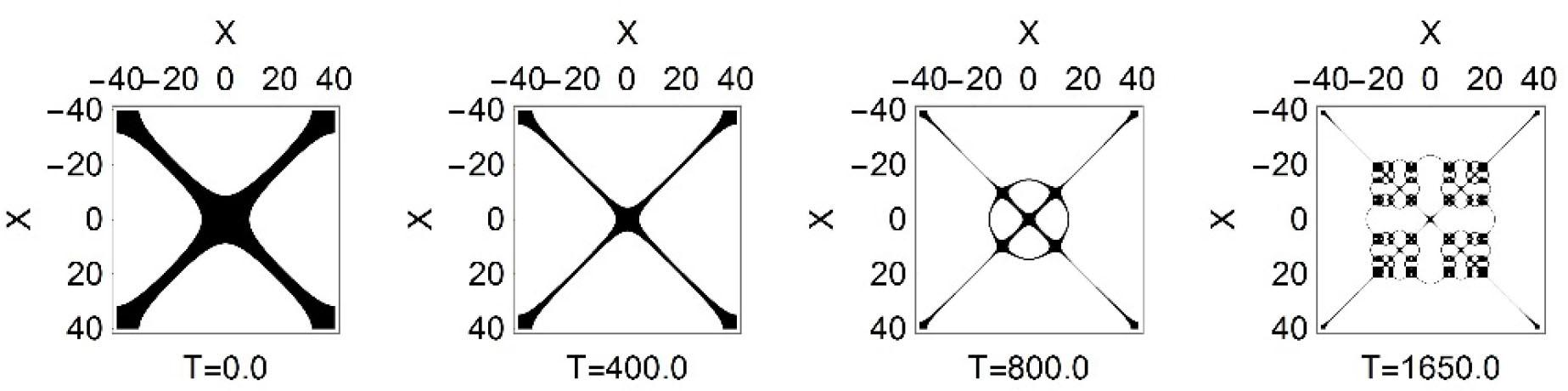Recurrence plot for a liquid film evolving on an IS-sub in zero gravity. Cut-off length, ɛ=0.0005. X, span-wise coordinate (dimensionless); T, time (dimensionless).
Visualizing Spatial Modes Through Recurrence Plots

To visualize the similarity in film dynamics and related spatial modes at different time instances with different substrates, a recurrence matrix was calculated and plotted. The recurrence matrix (Marwan et al., 2007), which is a sparse matrix, was calculated using Equation 8. $Rp=θ(ɛ−‖qj−qi‖)${R_p} = \theta \left( { \epsilon - \left\| {{q_j} - {q_i}} \right\|} \right)

Where ɛ is a properly chosen cut-off distance, qj and qi are states of the dynamical system at closely spaced, immediate spatial locations j and i, respectively, and θ is the unit step function. The ‖qjqi‖ is the Euclidean distance (or L-2 Norm) between states qj and qi. When the recurrence matrix was plotted as an array or matrix plot, a recurrence plot (RP) was available. This RP revealed all the times when the phase space trajectory of the dynamical system visited roughly (with proximity ɛ) the same position in the phase space. When a state recurred (in this case, when consecutive points on the film profile were within the cut-off distance of each other), the recurrence matrix was populated with “1.” Plotting this sparse matrix showed the locations of spatial recurrence (darkened positions correspond to “1”) and the presence of multiple modes through distortions in the diagonal or off-diagonal lines. Recurrence plots are used prominently in analyzing stochastic data such as machine tool vibrations, medical scans, and financial data and are an alternative to phase portraits. A phase portrait is not particularly useful to study film evolution because different points in the film evolve at different rates and to different amplitudes. The recurrence plot allows for a normalization, visualization, and analysis of spatially evolving modes at different time instances.

The evolution of a liquid film on the IS-sub in zero gravity is shown in Figure 3. A cut-off distance ɛ of 0.0005 signifies that the relative (spatial) difference of 0.05% between a current state and the previous state is treated as a recurrence or a recurrent state. Physically, this cut-off distance corresponds to ≈1 μm or less. This distance was nearly equal to rupture thickness in our simulations.

In contrast to Figure 3, the recurrence plot for a liquid film on the IP-sub in zero gravity is depicted in Figure 4. Higher spatial modes were damped when a porous substrate was used. This was seen as a lack of gaps in the diagonals and off-diagonal segments of the matrix plot. These gaps existed when an IP-sub was used (Figure 3), which in this case meant that there was a continuing development of destabilizing thermocapillary modes.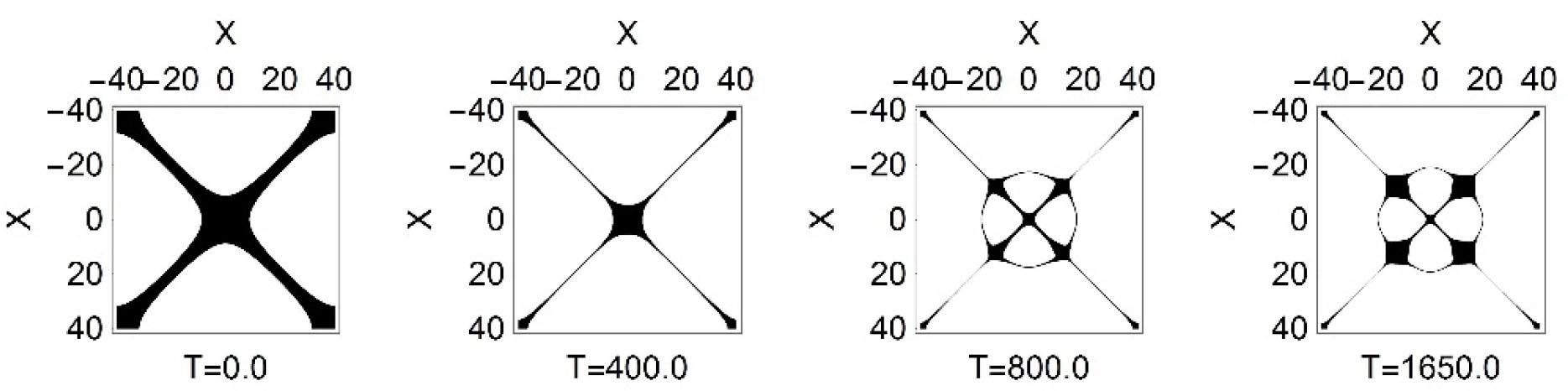Recurrence plot for a liquid film evolving on an IP-sub in zero gravity. Cut-off length, ɛ=0.0005. X, span-wise coordinate (dimensionless); T, time (dimensionless).

A difference in dynamics between liquid films on the two different substrates is visualized through a cross-recurrence plot (CRp, Equation 9) in Figure 5. In Equation 9, p and q are states of two different dynamical systems (i.e., film profile on a solid substrate versus that on a porous substrate) that are being compared for similarity at the same time. The cut-off distance used in Figure 5 was ɛ=0.0005. In other words, this cross-recurrence plot captured the nearness of film dynamics of a liquid film on IS-sub to the liquid film on IP-sub, at different spatial location, evolving in time, within 0.05% of each other. $CRp=θ(ɛ−‖p−q‖)$C{R_p} = \theta \left( { \epsilon - \left\| {p - q} \right\|} \right)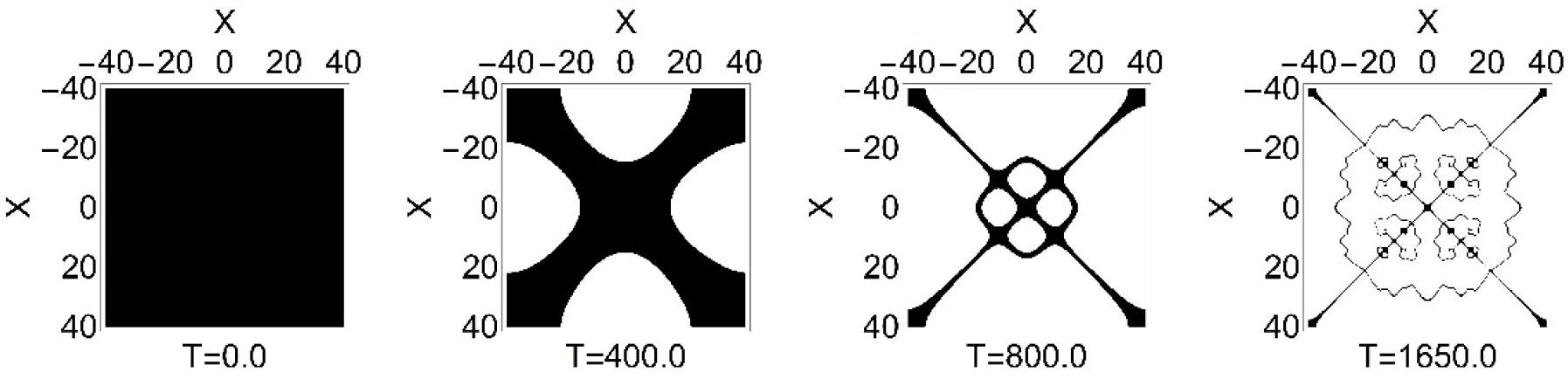Cross-recurrence plots (CRPs) of liquid film states for cut-off length, ɛ=0.0005. The CRPs visually compare the film dynamics on the IS-sub versus those on the IP-sub for a recurrence of states within 0.05% of each other. Because the IS-sub film ruptures at T=1650.0, CRP comparisons were made through T=1650.0. X, span-wise coordinate (dimensionless); T, time (dimensionless).

A larger value of the cut-off distance, ɛ=0.05, was used, and the closeness in film dynamics of a film on the IS-sub versus the film on the IP-sub is depicted in Figure 6. In other words, Figure 6 captured the recurrence of spatial-temporal states within 5% of each other. To quantify this closeness in dynamics, a rate of recurrence (RR%), which is one of the many recurrence quantification parameters (Marwan et al., 2007) was calculated and tabulated in Table 1. The rate of recurrence is simply the percentage of ‘1’ in the sparse recurrence matrix. A greater percentage of ‘1’ suggests a greater rate or percentage of recurrence.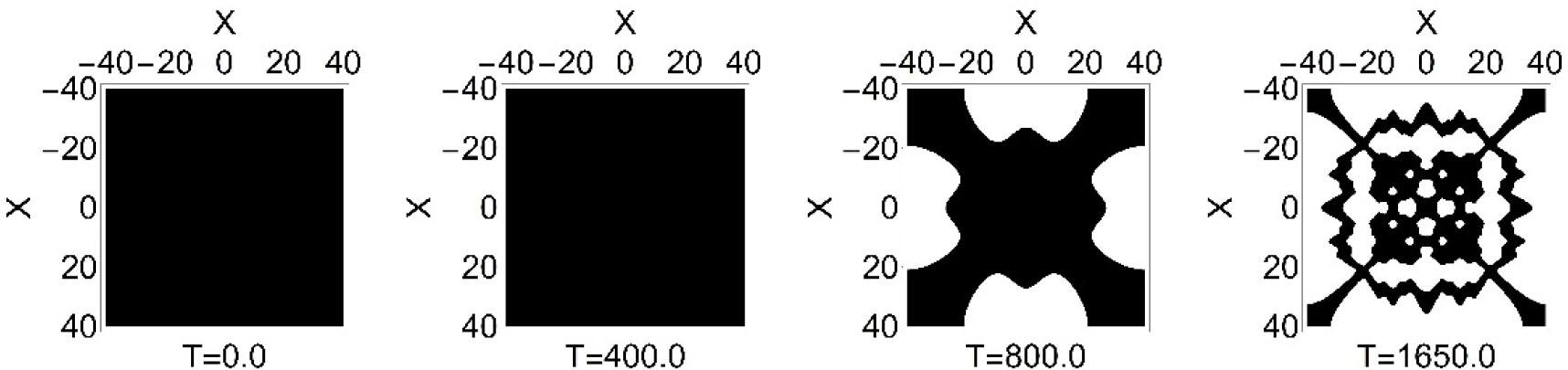Cross-recurrence plots (CRPs) of liquid film states for cut-off length, ɛ=0.05. The CRPs visually compare the film dynamics on the IS-sub versus those on the IP-sub for a recurrence of states within 5% of each other. X, span-wise coordinate (dimensionless); T, time (dimensionless).

Rates of recurrence comparing similarity of liquid films dynamics on IS-sub vs. IP-sub. Two different cut-off distances, ɛ, were used to describe the similarity between dynamics of liquid films. IS-sub, isothermal solid substrate; IP-sub, isothermal porous substrate.

Liquid film on IS-sub vs. IP-sub with ɛ=0.05Liquid film on IS-sub vs. IP-sub with ɛ=0.0005
Time (non-dimensional)Recurrence Rate (RR%)SignificanceTime (non-dimensional)Recurrence Rate (RR%)Significance
0.0100.0Similar dynamics0.0100.0Similar dynamics
400.0100.0Similar dynamics400.033.66Dynamics within 33.66% of each other
800.045.94Dynamics within 45.94% of each other800.06.86Dynamics within 6.86% of each other
1650.0 (time rupture of film on IS-sub)39.79Dynamics within 39.79% of each other1650.05.29Dynamics within 5.29% of each other
DISCUSSION

In this short communication, numerical simulations were conducted for a Newtonian liquid film on an isothermal solid (IS-sub) and isothermal porous substrate (IP-sub), in zero gravity. Here, the film was flat and parallel to the substrate. The porous substrate was treated as having one-dimensional depth that was fully saturated with the same fluid as that of the liquid film, and the substrate was sufficient to store the entire volume of the liquid film that rested on it. A nonlinear evolution equation derived using a long wave expansion was solved as an initial value problem with periodic boundary conditions. The liquid film was under the influence of stabilizing surface tension and destabilizing thermocapillarity, with zero gravitational acceleration. With the IS-sub, the film ruptured through the creation and growth of thermocapillary modes and concomitant local thinning.

Next, a weakly porous substrate was chosen to ensure that the fastest growing wavelength stayed nearly the same as that for the non-porous substrate. We observed that this had a damping effect on spatial thermocapillary modes and prolonged film lifespan without rupture. Rupture of the film on the isothermal porous substrate was not observed in the simulated time frame.

Recurrence plots were used to analyze the temporal evolution of spatial thermocapillary modes, with and without a porous substrate. An isothermal porous substrate led to the damping of higher spatial modes. Cross-recurrence plots (CRPs) were used to visualize a difference in states between a liquid film on an isothermal solid substrate and an isothermal porous substrate. A recurrence rate (RR%) measuring the nearness of dynamics of the film states with different substrates revealed that with a weakly porous substrate, the film dynamics—although similar at early stages of evolution—led to different states at later stages.

Future work will include studies of zero gravity liquid film/coating pattern fidelity using recurrence plots and recurrence quantification. Windowing of recurrence plots will be used to quantify temporal evolution of spatial points of interest.

#### Rates of recurrence comparing similarity of liquid films dynamics on IS-sub vs. IP-sub. Two different cut-off distances, ɛ, were used to describe the similarity between dynamics of liquid films. IS-sub, isothermal solid substrate; IP-sub, isothermal porous substrate.

Liquid film on IS-sub vs. IP-sub with ɛ=0.05Liquid film on IS-sub vs. IP-sub with ɛ=0.0005
Time (non-dimensional)Recurrence Rate (RR%)SignificanceTime (non-dimensional)Recurrence Rate (RR%)Significance
0.0100.0Similar dynamics0.0100.0Similar dynamics
400.0100.0Similar dynamics400.033.66Dynamics within 33.66% of each other
800.045.94Dynamics within 45.94% of each other800.06.86Dynamics within 6.86% of each other
1650.0 (time rupture of film on IS-sub)39.79Dynamics within 39.79% of each other1650.05.29Dynamics within 5.29% of each other

Ajaev VS (2012) Interfacial Fluid Mechanics: A Mathematical Modeling Approach, Boston: Springer.AjaevVS2012Interfacial Fluid Mechanics: A Mathematical Modeling ApproachBostonSpringer10.1007/978-1-4614-1341-7Search in Google Scholar

Burelbach JP, Bankoff SG, Davis SH (1988) Nonlinear stability of evaporating/condensing liquid films. Journal of Fluid Mechanics195: 463–494BurelbachJPBankoffSGDavisSH1988Nonlinear stability of evaporating/condensing liquid filmsJournal of Fluid Mechanics19546349410.1017/S0022112088002484Search in Google Scholar

Craster RV, Matar OK (2009) Dynamics and stability of thin liquid films. Reviews of Modern Physics81(3): 1131CrasterRVMatarOK2009Dynamics and stability of thin liquid filmsReviews of Modern Physics813113110.1103/RevModPhys.81.1131Search in Google Scholar

Davis, SH, Hocking LM (1999) Spreading and imbibition of viscous liquid on a porous base. Physics of Fluids11(1): 48–57DavisSHHockingLM1999Spreading and imbibition of viscous liquid on a porous basePhysics of Fluids111485710.1063/1.869901Search in Google Scholar

Liu Y, Zheng Z, Stone HA (2017) The influence of capillary effects on the drainage of a viscous gravity current into a deep porous medium. Journal of Fluid Mechanics817: 514–559LiuYZhengZStoneHA2017The influence of capillary effects on the drainage of a viscous gravity current into a deep porous mediumJournal of Fluid Mechanics81751455910.1017/jfm.2017.125Search in Google Scholar

Marwan N, Romano MC, Thiel M, Kurths J (2007) Recurrence plots for the analysis of complex systems. Physics Reports438(5): 237–329MarwanNRomanoMCThielMKurthsJ2007Recurrence plots for the analysis of complex systemsPhysics Reports438523732910.1016/j.physrep.2006.11.001Search in Google Scholar

Momoniat E, Ravindran R, Roy S (2010) The influence of slot injection/suction on the spreading of a thin film under gravity and surface tension. Acta Mechanica211(1): 61–71MomoniatERavindranRRoyS2010The influence of slot injection/suction on the spreading of a thin film under gravity and surface tensionActa Mechanica2111617110.1007/s00707-009-0215-ySearch in Google Scholar

Narendranath AD, Hermanson JC, Kolkka RW, Struthers AA, Allen JS (2014) The effect of gravity on the stability of an evaporating liquid film. Microgravity Science and Technology26(3): 189–199NarendranathADHermansonJCKolkkaRWStruthersAAAllenJS2014The effect of gravity on the stability of an evaporating liquid filmMicrogravity Science and Technology26318919910.1007/s12217-014-9395-8Search in Google Scholar

Oron A (2000) Three-dimensional nonlinear dynamics of thin liquid films. Physical Review Letters85(10): 2108OronA2000Three-dimensional nonlinear dynamics of thin liquid filmsPhysical Review Letters8510210810.1103/PhysRevLett.85.2108Search in Google Scholar

Oron A, Davis SH, Bankoff SG (1997) Long-scale evolution of thin liquid films. Reviews of Modern Physics69(3): 931OronADavisSHBankoffSG1997Long-scale evolution of thin liquid filmsReviews of Modern Physics69393110.1103/RevModPhys.69.931Search in Google Scholar

Thompson AB, Tseluiko D, Papageorgiou DT (2016) Falling liquid films with blowing and suction. Journal of Fluid Mechanics787: 292–330ThompsonABTseluikoDPapageorgiouDT2016Falling liquid films with blowing and suctionJournal of Fluid Mechanics78729233010.1017/jfm.2015.683Search in Google Scholar